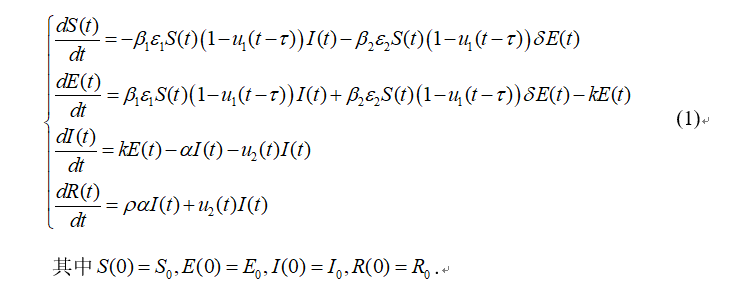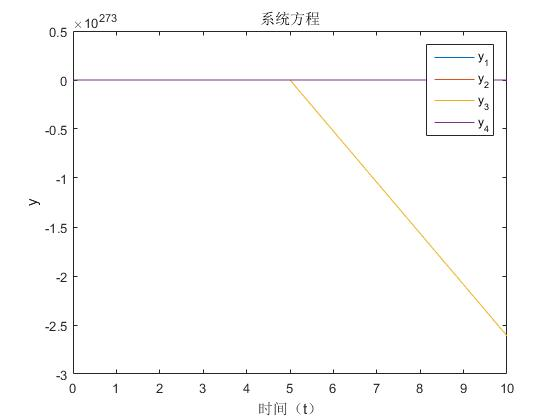# 求大佬帮我看一下我的四阶龙格库塔法求解常微分方程组的MATLAB程序对吗1.  function f=myfun(t,y,beta1,beta2,epsilong1,epsilong2,delta,u1,u2,k,alpha,p) f(1)=-beta1.*epsilong1.*y(1).*(1-u1).*y(3)-beta2.*epsilong2.*y(1).*(1-u1).*delta.*y(2); f(2)=beta1.*epsilong1.*y(1).*(1-u1).*y(3)-beta2.*epsilong2.*y(1).*(1-u1).*delta.*y(2)-k*y(2); f(3)=k.*y(2)-alpha.*y(3)-u2.*y(3); f(4)=p.*alpha.*y(3)+u2.*y(3); f=f( : );  这是我的函数m文件
1.  function [ t, y]=RK4(myfun,tspan,y0,h) t=tspan(1):h:tspan(2); y=zeros(length(y0),length(t)); y(:,1)=y0(:); for n=1:(length(t)-1) k1=feval(myfun,t(n),y(:,n)); k2=feval(myfun,t(n)+h/2,y(:,n)+h/2*k1); k3=feval(myfun,t(n)+h/2,y(:,n)+h/2*k2); k4=feval(myfun,t(n+1),y(:,n)+h*k3); y(:,n+1)=y(:,n)+(h/6).*(k1+2*k2+2*k3+k4); end  这是四阶龙格库塔的程序文件 3.  clear; clc; tspan=[0 300]; y0=[1000000 0 1 0]; % 初值 beta1=0.8; beta2=0.5; epsilong1=0.5; epsilong2=0.2; delta=0.5; u1=0.7; u2=0.03; k=0.526; alpha=0.244; p=0.93; [t, y]=RK4(@(t,y)myfun(t,y,beta1,beta2,epsilong1,epsilong2,delta,u1,u2,k,alpha,p),tspan,y0,5); plot(t,y); title('系统方程');xlabel('时间（t）');ylabel('y'); legend('y_1','y_2','y_3','y_4',2);  这是最后的程序，上面附了一些初值，最后得出的结果是这样的，这是线性的吗，感觉很懵逼啊，而且y的值怎么会出现负的
• 点赞
• 写回答
• 关注问题
• 收藏
• 复制链接分享
• 邀请回答